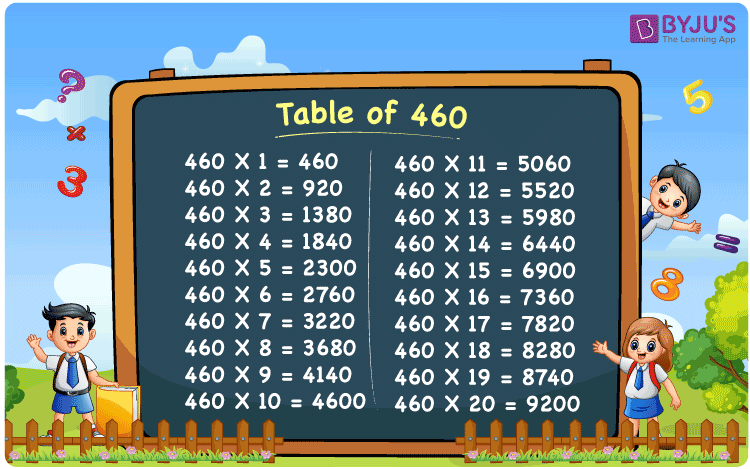Checkout JEE MAINS 2022 Question Paper Analysis : Checkout JEE MAINS 2022 Question Paper Analysis :

# Table of 460

The table of 460 is offered here to assist students in checking the table of 460 values up to 20 times. In this article, the 460 times table is included in a tabular format. You will also get the multiplication table for 460 by downloading the PDF from our page. The table of 460 chart can be used as a flashcard by students, which will help them learn and remember the values quickly.

## Table of 460 Chart## What is the 460 Times Table?

The 460 times table is also known as the multiplication table of 460, which is obtained either by multiplying 460 with natural numbers or by repeatedly adding 460 to itself for the specified number of times. The below table shows the multiplication and repeated addition of the 460 times table.

 460×1 = 460 460 460×2 = 920 460 + 460 = 920 460×3 = 1380 460 + 460 + 460 = 1380 460×4 = 1840 460 + 460 + 460 + 460 = 1840 460×5 = 2300 460 + 460 + 460 + 460 + 460 = 2300 460×6 = 2760 460 + 460 + 460 + 460 + 460 + 460 = 2760 460×7 = 3220 460 + 460 + 460 + 460 + 460 + 460 + 460 = 3220 460×8 = 3680 460 + 460 + 460 + 460 + 460 + 460 + 460 + 460 = 3680 460×9 = 4140 460 + 460 + 460 + 460 + 460 + 460 + 460 + 460 + 460 = 4140 460×10 = 4600 460 + 460 + 460 + 460 + 460 + 460 + 460 + 460 + 460 + 460 = 4600

## Multiplication Table of 460

The table below shows the first 20 multiples of 460.

 460 × 1 = 460 460 × 2 = 920 460 × 3 = 1380 460 × 4 = 1840 460 × 5 = 2300 460 × 6 = 2760 460 × 7 = 3220 460 × 8 = 3680 460 × 9 = 4140 460 × 10 = 4600 460 × 11 = 5060 460 × 12 = 5520 460 × 13 = 5980 460 × 14 = 6440 460 × 15 = 6900 460 × 16 = 7360 460 × 17 = 7820 460 × 18 = 8280 460 × 19 = 8740 460 × 20 = 9200

## Solved Example on the Table of 460

Example:

What is 100 less than the 12th multiple of 460?

Solution:

Using the multiplication table of 460,

The 12th multiple of 460 is 5520.

I.e., 12 × 460 = 5520

Therefore, 100 less than the 12th multiple of 460 = 5520 – 100 = 5420.

## Frequently Asked Questions on the Table of 460

### What is the table of 460?

460×1 = 460, 460×2 = 920, 460×3 = 1380, 460×4 = 1840, 460×5 = 2300, 460×6 = 2760, 460×7 = 3220, 460×8 = 3680, 460×9 = 4140, 460×10 = 4600.

### How many times does 460 equal 9200?

20 times 460 equals 9200.

### What is 460 times 9?

460 times 9 is 4140. I.e., 460 × 9 = 4140.